$\newcommand{\NN}{\mathbb{N}} \newcommand{\CC}{\mathbb{C}} \newcommand{\GG}{\mathbb{G}} \newcommand{\LL}{\mathbb{L}} \newcommand{\PP}{\mathbb{P}} \newcommand{\QQ}{\mathbb{Q}} \newcommand{\RR}{\mathbb{R}} \newcommand{\VV}{\mathbb{V}} \newcommand{\ZZ}{\mathbb{Z}} \newcommand{\FF}{\mathbb{F}} \newcommand{\KK}{\mathbb{K}} \newcommand{\UU}{\mathbb{U}} \newcommand{\EE}{\mathbb{E}} \newcommand{\Aa}{\mathcal{A}} \newcommand{\Bb}{\mathcal{B}} \newcommand{\Cc}{\mathcal{C}} \newcommand{\Dd}{\mathcal{D}} \newcommand{\Ee}{\mathcal{E}} \newcommand{\Ff}{\mathcal{F}} \newcommand{\Gg}{\mathcal{G}} \newcommand{\Hh}{\mathcal{H}} \newcommand{\Ii}{\mathcal{I}} \newcommand{\Jj}{\mathcal{J}} \newcommand{\Kk}{\mathcal{K}} \newcommand{\Ll}{\mathcal{L}} \newcommand{\Mm}{\mathcal{M}} \newcommand{\Nn}{\mathcal{N}} \newcommand{\Oo}{\mathcal{O}} \newcommand{\Pp}{\mathcal{P}} \newcommand{\Qq}{\mathcal{Q}} \newcommand{\Rr}{\mathcal{R}} \newcommand{\Ss}{\mathcal{S}} \newcommand{\Tt}{\mathcal{T}} \newcommand{\Uu}{\mathcal{U}} \newcommand{\Vv}{\mathcal{V}} \newcommand{\Ww}{\mathcal{W}} \newcommand{\Xx}{\mathcal{X}} \newcommand{\Yy}{\mathcal{Y}} \newcommand{\Zz}{\mathcal{Z}} \newcommand{\al}{\alpha} \newcommand{\la}{\lambda} \newcommand{\ga}{\gamma} \newcommand{\Ga}{\Gamma} \newcommand{\La}{\Lambda} \newcommand{\Si}{\Sigma} \newcommand{\si}{\sigma} \newcommand{\be}{\beta} \newcommand{\de}{\delta} \newcommand{\De}{\Delta} \renewcommand{\phi}{\varphi} \renewcommand{\th}{\theta} \newcommand{\om}{\omega} \newcommand{\Om}{\Omega} \renewcommand{\epsilon}{\varepsilon} \newcommand{\Calpha}{\mathrm{C}^\al} \newcommand{\Cbeta}{\mathrm{C}^\be} \newcommand{\Cal}{\text{C}^\al} \newcommand{\Cdeux}{\text{C}^{2}} \newcommand{\Cun}{\text{C}^{1}} \newcommand{\Calt}{\text{C}^{#1}} \newcommand{\lun}{\ell^1} \newcommand{\ldeux}{\ell^2} \newcommand{\linf}{\ell^\infty} \newcommand{\ldeuxj}{{\ldeux_j}} \newcommand{\Lun}{\text{\upshape L}^1} \newcommand{\Ldeux}{\text{\upshape L}^2} \newcommand{\Lp}{\text{\upshape L}^p} \newcommand{\Lq}{\text{\upshape L}^q} \newcommand{\Linf}{\text{\upshape L}^\infty} \newcommand{\lzero}{\ell^0} \newcommand{\lp}{\ell^p} \renewcommand{\d}{\ins{d}} \newcommand{\Grad}{\text{Grad}} \newcommand{\grad}{\text{grad}} \renewcommand{\div}{\text{div}} \newcommand{\diag}{\text{diag}} \newcommand{\pd}{ \frac{ \partial #1}{\partial #2} } \newcommand{\pdd}{ \frac{ \partial^2 #1}{\partial #2^2} } \newcommand{\dotp}{\langle #1,\,#2\rangle} \newcommand{\norm}{|\!| #1 |\!|} \newcommand{\normi}{\norm{#1}_{\infty}} \newcommand{\normu}{\norm{#1}_{1}} \newcommand{\normz}{\norm{#1}_{0}} \newcommand{\abs}{\vert #1 \vert} \newcommand{\argmin}{\text{argmin}} \newcommand{\argmax}{\text{argmax}} \newcommand{\uargmin}{\underset{#1}{\argmin}\;} \newcommand{\uargmax}{\underset{#1}{\argmax}\;} \newcommand{\umin}{\underset{#1}{\min}\;} \newcommand{\umax}{\underset{#1}{\max}\;} \newcommand{\pa}{\left( #1 \right)} \newcommand{\choice}{ \left\{ \begin{array}{l} #1 \end{array} \right. } \newcommand{\enscond}{ \left\{ #1 \;:\; #2 \right\} } \newcommand{\qandq}{ \quad \text{and} \quad } \newcommand{\qqandqq}{ \qquad \text{and} \qquad } \newcommand{\qifq}{ \quad \text{if} \quad } \newcommand{\qqifqq}{ \qquad \text{if} \qquad } \newcommand{\qwhereq}{ \quad \text{where} \quad } \newcommand{\qqwhereqq}{ \qquad \text{where} \qquad } \newcommand{\qwithq}{ \quad \text{with} \quad } \newcommand{\qqwithqq}{ \qquad \text{with} \qquad } \newcommand{\qforq}{ \quad \text{for} \quad } \newcommand{\qqforqq}{ \qquad \text{for} \qquad } \newcommand{\qqsinceqq}{ \qquad \text{since} \qquad } \newcommand{\qsinceq}{ \quad \text{since} \quad } \newcommand{\qarrq}{\quad\Longrightarrow\quad} \newcommand{\qqarrqq}{\quad\Longrightarrow\quad} \newcommand{\qiffq}{\quad\Longleftrightarrow\quad} \newcommand{\qqiffqq}{\qquad\Longleftrightarrow\qquad} \newcommand{\qsubjq}{ \quad \text{subject to} \quad } \newcommand{\qqsubjqq}{ \qquad \text{subject to} \qquad }$

Image Deconvolution using Sparse Regularization

# Image Deconvolution using Sparse Regularization

This numerical tour explores the use of sparsity regularization to perform image deconvolution.

## Installing toolboxes and setting up the path.

You need to download the following files: signal toolbox and general toolbox.

You need to unzip these toolboxes in your working directory, so that you have toolbox_signal and toolbox_general in your directory.

For Scilab user: you must replace the Matlab comment '%' by its Scilab counterpart '//'.

Recommandation: You should create a text file named for instance numericaltour.sce (in Scilab) or numericaltour.m (in Matlab) to write all the Scilab/Matlab command you want to execute. Then, simply run exec('numericaltour.sce'); (in Scilab) or numericaltour; (in Matlab) to run the commands.

Execute this line only if you are using Matlab.

getd = @(p)path(p,path); % scilab users must *not* execute this


Then you can add the toolboxes to the path.

getd('toolbox_signal/');
getd('toolbox_general/');


## Sparse Regularization

This tour consider measurements $$y=\Phi f_0 + w$$ where $$\Phi$$ is a convolution $$\Phi f = h \star f$$ and $$w$$ is an additive noise.

This tour is focussed on using sparsity to recover an image from the measurements $$y$$. It consider a synthesis-based regularization, that compute a sparse set of coefficients $$(a_m^{\star})_m$$ in a frame $$\Psi = (\psi_m)_m$$ that solves $a^{\star} \in \text{argmin}_a \: \frac{1}{2}\|y-\Phi \Psi a\|^2 + \lambda J(a)$

where $$\lambda$$ should be adapted to the noise level $$\|w\|$$

Here we used the notation $\Psi a = \sum_m a_m \psi_m$ to indicate the reconstruction operator, and $$J(a)$$ is the $$\ell^1$$ sparsity prior $J(a)=\sum_m \|a_m\|.$

## Image Blurring

Deconvolution corresponds to removing a blur from an image. We use here a Gaussian blur.

Parameters for the tour: width of the kernel (in pixel) and noise level.

setting = 1;
switch setting
case 1
% difficult
s = 3;
sigma = .02;
case 2
% easy
s = 1.2;
sigma = .02;
end


First we load the image to be processed.

n = 128*2;
name = 'lena';
name = 'boat';
name = 'mri';
f0 = rescale(crop(f0,n));


Display it.

clf;
imageplot(f0);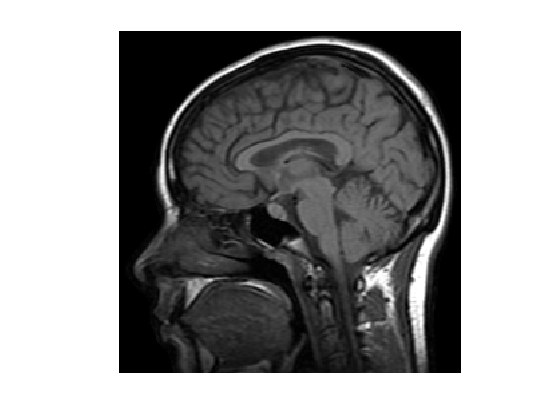We build a convolution kernel. Since we are going to use Fourier to compute the convolution, we set the center of the kernel in the (1,1) pixel location.

Kernel.

x = [0:n/2-1, -n/2:-1];
[Y,X] = meshgrid(x,x);
h = exp( (-X.^2-Y.^2)/(2*s^2) );
h = h/sum(h(:));


Useful for later : the Fourier transform (should be real because of symmetry).

hF = real(fft2(h));


Display the kernel $$h$$ and its transform $$\hat h$$. We use fftshift to center the filter for display.

clf;
imageplot(fftshift(h), 'Filter', 1,2,1);
imageplot(fftshift(hF), 'Fourier transform', 1,2,2);We use this short hand for the filtering. Scilab user should define a function in a separate file to perform this. Note that this is a symmetric operator.

Phi = @(x)real(ifft2(fft2(x).*hF));


Apply the filter.

y0 = Phi(f0);


Display the filtered observation.

clf;
imageplot(f0, 'Image f0', 1,2,1);
imageplot(y0, 'Observation without noise', 1,2,2);Add some noise of variance $$\sigma^2$$, to obtain $$y=\Phi f_0 + w = f_0 \star h + w$$.

y = y0 + randn(n,n)*sigma;


Display.

clf;
imageplot(y0, 'Observation without noise', 1,2,1);
imageplot(clamp(y), 'Observation with noise', 1,2,2);## Soft Thresholding in a Basis

The soft thresholding operator is at the heart of $$\ell^1$$ minimization schemes. It can be applied to coefficients $$a$$, or to an image $$f$$ in an ortho-basis.

The soft thresholding is a 1-D functional that shrinks the value of coefficients. $s_T(u)=\max(0,1-T/|u|)u$

Define a shortcut for this soft thresholding 1-D functional.

SoftThresh = @(x,T)x.*max( 0, 1-T./max(abs(x),1e-10) );


Display a curve of the 1D soft thresholding.

clf;
T = linspace(-1,1,1000);
plot( T, SoftThresh(T,.5) );
axis('equal');Note that the function SoftThresh can also be applied to vector (because of Matlab/Scilab vectorialized computation), which defines an operator on coefficients: $S_T(a) = ( s_T(a_m) )_m.$

In the next section, we use an orthogonal wavelet basis $$\Psi$$.

We set the parameters of the wavelet transform.

Jmax = log2(n)-1;
Jmin = Jmax-3;


Shortcut for $$\Psi$$ and $$\Psi^*$$ in the orthogonal case.

options.ti = 0; % use orthogonality.
Psi = @(a)perform_wavelet_transf(a, Jmin, -1,options);
PsiS = @(f)perform_wavelet_transf(f, Jmin, +1,options);


The soft thresholding opterator in the basis $$\Psi$$ is defined as $S_T^\Psi(f) = \sum_m s_T( \langle f,\psi_m \rangle ) \psi_m$

It thus corresponds to applying the transform $$\Psi^*$$, thresholding the coefficients using $$S_T$$ and then undoing the transform using $$\Psi$$. $S_T^\Psi(f) = \Psi \circ S_T \circ \Psi^*$

SoftThreshPsi = @(f,T)Psi(SoftThresh(PsiS(f),T));


This soft thresholding corresponds to a denoising operator.

clf;
imageplot( clamp(SoftThreshPsi(f0,.1)) );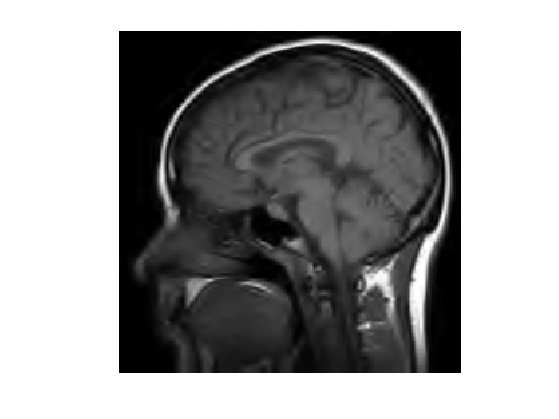## Deconvolution using Orthogonal Wavelet Sparsity

If $$\Psi$$ is an orthogonal basis, a change of variable shows that the synthesis prior is also an analysis prior, that reads $f^{\star} \in \text{argmin}_f \: E(f) = \frac{1}{2}\|y-\Phi f\|^2 + \lambda \sum_m \|\langle f,\psi_m \rangle\|.$

To solve this non-smooth optimization problem, one can use forward-backward splitting, also known as iterative soft thresholding.

It computes a series of images $$f^{(\ell)}$$ defined as $f^{(\ell+1)} = S_{\tau\lambda}^{\Psi}( f^{(\ell)} - \tau \Phi^{*} (\Phi f^{(\ell)} - y) )$

Set up the value of the threshold.

lambda = .02;


In our setting, since $$h$$ is symmetric, one has $$\Phi^* f = \Phi f = f \star h$$.

For $$f^{(\ell)}$$ to converge to a solution of the problem, the gradient step size should be chosen as $\tau < \frac{2}{\|\Phi^* \Phi\|}$

Since the filtering is an operator of norm 1, this must be smaller than 2.

tau = 1.5;


Number of iterations.

niter = 100;


Initialize the solution.

fSpars = y;


First step: perform one step of gradient descent of the energy $$\|y-f\star h\|^2$$.

fSpars = fSpars + tau * Phi( y-Phi(fSpars) );


Second step: denoise the solution by thresholding.

fSpars = SoftThreshPsi( fSpars, lambda*tau );


Exercice 1: (check the solution) Perform the iterative soft thresholding. Monitor the decay of the energy $$E$$ you are minimizing.

exo1;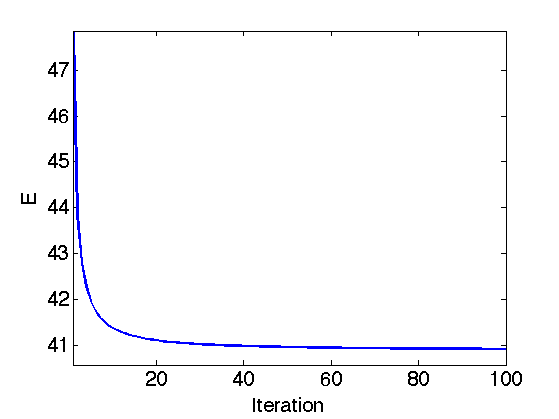Display the result.

clf;
imageplot(clamp(fSpars), ['Sparsity deconvolution, SNR=' num2str(snr(f0,fSpars),3) 'dB']);Exercice 2: (check the solution) Try to find the best threshold $$\lambda$$. To this end, perform a lot of iterations, and progressively decay the threshold $$\lambda$$ during the iterations. Record the best result in fBestOrtho.

exo2;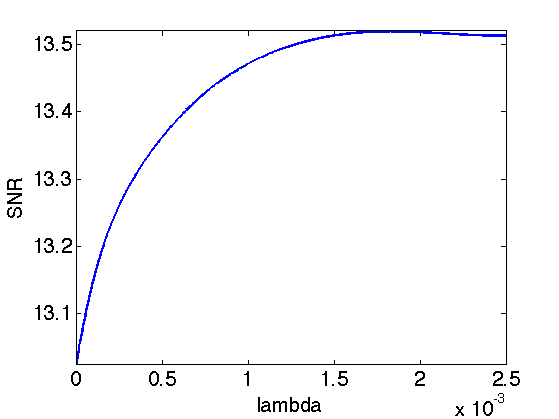Display the result.

clf;
imageplot(clamp(fBestOrtho), ['Sparsity deconvolution, SNR=' num2str(snr(f0,fBestOrtho),3) 'dB']);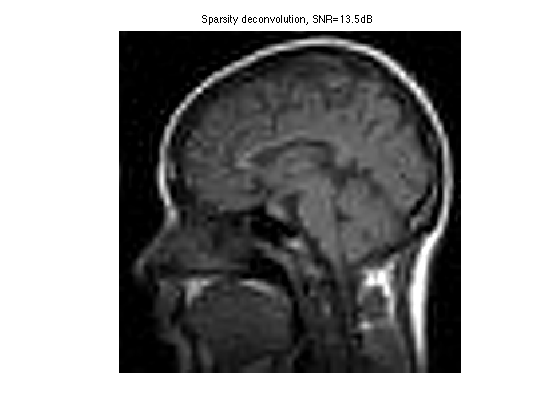## Deconvolution using Translation Invariant Wavelet Sparsity

Orthogonal sparsity performs a poor regularization because of the lack of translation invariance. This regularization is enhanced by considering $$\Psi$$ as a redundant tight frame of translation invariant wavelets.

One thus looks for optimal coefficients $$a^\star$$ that solves $a^{\star} \in \text{argmin}_a \: \frac{1}{2}\|y-\Phi \Psi a\|^2 + \lambda J(a)$

One should be careful that because of the redundancy of the wavelet tight frame, one should use a weighted $$\ell^1$$ norm, where each coefficient is divided by the number of redundancy at each scale. $J(a) = \sum_{j,k} u_j \|a_{j,k}\|$

where the wavelet coefficients $$a_{m} = a_{j,k}$$ are indexed by the scale (and orientation) $$j$$ and the location $$k$$.

Compute the scaling factor (inverse of the redundancy).

J = Jmax-Jmin+1;
u = [4^(-J) 4.^(-floor(J+2/3:-1/3:1)) ];


Value of the regularization parameter.

lambda = .01;


Shortcut for the wavelet transform. Important: Note that PsiS is the shortcut for $$\Psi^*$$, but Psi is the shortcut for $$\Xi = (\Psi^*)^+$$ that satisfy $$\Xi \Phi^* f = f$$.

options.ti = 1; % use translation invariance
Psi = @(a)perform_wavelet_transf(a, Jmin, -1,options);
PsiS = @(f)perform_wavelet_transf(f, Jmin, +1,options);


The forward-backward algorithm now compute a series of wavelet coefficients $$a^{(\ell)}$$ computed as $a^{(\ell+1)} = S_{\tau\lambda}( a^{(\ell)} + \Psi^*\Phi( y - \Phi\Xi a^{(\ell)} ) ).$

The soft thresholding is defined as: $\forall m, \quad S_T(a)_m = \max(0, 1-T/\|a_m\|)a_m.$

The step size should satisfy: $\tau < \frac{2}{\|\Phi\Psi\|^2}.$

tau = 1.5;


Initialize the wavelet coefficients with those of the observations.

a = PsiS(y);


a = a + tau * PsiS( Phi( y-Phi(Psi(a)) ) );


Soft threshold.

a = SoftThresh( a, lambda*tau );


Important: keep in mind that the prior $$J(a)$$ is a weighted $$\ell^1$$ norm, it should thus be computed this way:

U = repmat( reshape(u,[1 1 length(u)]), [n n 1] );
Ja = sum(sum(sum( abs(a.*U) )));


Exercice 3: (check the solution) Perform the iterative soft thresholding. Monitor the decay of the energy.

exo3;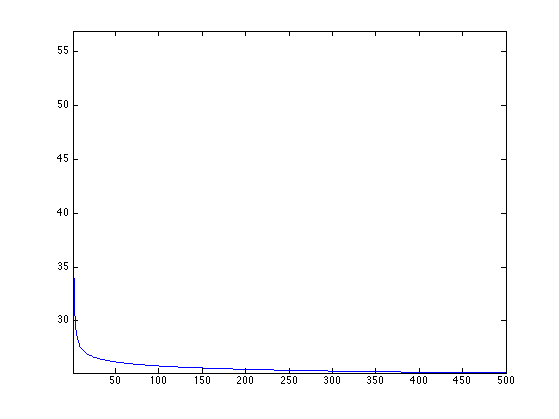Perform the reconstruction.

fTI = Psi(a);


Display the result.

clf;
imageplot(fTI);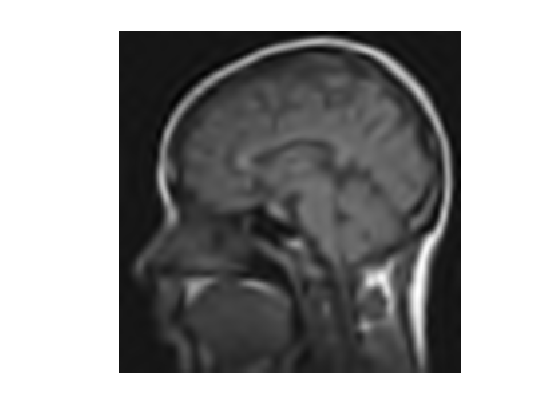Exercice 4: (check the solution) Compute the optimal value of $$\lambda$$, and record the optimal reconstruction fBestTI.

exo4;Display the result.

clf;
imageplot(clamp(fBestTI), ['Sparsity deconvolution TI, SNR=' num2str(snr(f0,fBestTI),3) 'dB']);Exercice 5: (check the solution) Compare with the result of TV regularization, record the optimal TV result in fBestTV.

exo5;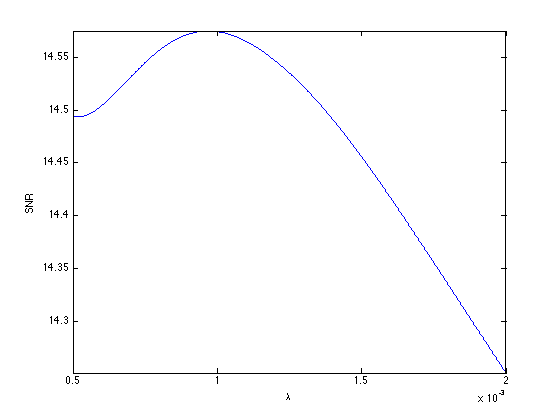Display the result.

clf;
imageplot(clamp(fBestTV), ['TV deconvolution, SNR=' num2str(snr(f0,fBestTV),3) 'dB']);